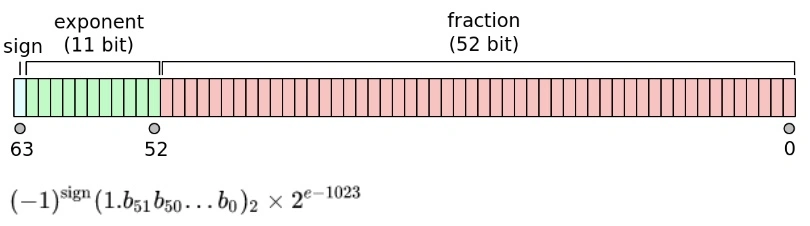# One post tagged with "currency"

View All Tags

## Intro​

Calculating with money can be tricky if not taken proper precautions. Some might be tempted to use float representation for calculating with currency values. That is problematic because of possible rounding errors.

## Finite accuracy of representation​

Floating points are represented like thisNot every number can be represented with a finite number of decimal places

0.01 —> 0.0000011001100110011…

Taking 17 places of the above results in 0.010000000000000001

Consider the following code snipet that shows the missing accuracy

``func main() {  var n float64 = 0  for i := 0; i < 1000; i++ {    n += .01  }  fmt.Println(n)}``

Result: 9.999999999999831

## Money computations​

They can't be done with floating-point as it would inevitably lead to rounding errors.

Even the following packages are problematic:

github.com/shopspring/decimal

github.com/Rhymond/go-money

``a := decimal.NewFromInt(2)b := decimal.NewFromFloat(300.99)c := a.Mul(b)d := c.Div(decimal.NewFromInt(3))``

## Solution​

Use Int by representing money in cents:

• 10.99 -> 1099 (cents)
• 10.9900 -> 109900 (4 digit tax)

## Conclusion​

Division is a problem!

1/3 - > 0.33333333… Correct way: 0.33, 0.33, 0.34

When doing money calculations one should avoid division as it inevitably leads to loss of accuracy. When dividing make sure to round to cent and deal with diffs.

Division by 10^k is ok as long as we are inside of the range of the data type.